Function Repository Resource:

# CurvedSpacetimeTriangulation

Produce causal graphs by dynamically triangulating a specified region of a (potentially curved) spacetime

Contributed by: Jonathan Gorard
 ResourceFunction["CurvedSpacetimeTriangulation"][cond,{x1,…,xn},meas] produces a dynamical triangulation of a (potentially curved) spacetime region that satisfies the conditions cond. ResourceFunction["CurvedSpacetimeTriangulation"][cond,{x1,…,xn},{{xmin,xmax},…},meas] restricts the triangulation to the bounds {xmin,xmax}×…. ResourceFunction["CurvedSpacetimeTriangulation"][cond,{x1,…,xn},meas,"prop"] gives the property "prop" for the specified triangulation. ResourceFunction["CurvedSpacetimeTriangulation"][cond,{x1,…,xn},{{xmin,xmax},…},meas,"prop"] gives the property "prop" for the specified restricted triangulation.

## Details

When no "prop" is given, ResourceFunction["CurvedSpacetimeTriangulation"] returns a SpacetimeTriangulation object by default.
By default, the region is restricted to the bounds {{-1,1},}.
Triangulations are constructed using causal dynamical triangulation (CDT) techniques, which generalize the Euclidean dynamical triangulation (EDT) techniques implemented by functions like MeshRegion and DiscretizeRegion.
Currently, triangulations can only be constructed for 0+1-, 1+1- and 2+1-dimensional spacetimes using ResourceFunction["CurvedSpacetimeTriangulation"].
Causal edges are added in accordance with the standard causal partial-order relation on spacetime (as determined using the usual form of the spacetime line element) and are transitively reduced by default. Causal edges may optionally be added only to those pairs of events that are connected within the triangulation (e.g. "TriangulatedCausalGraph") or otherwise to all pairs of events that are causally related regardless of the triangulation (e.g. "CausalGraph").
ResourceFunction["CurvedSpacetimeTriangulation"][cond,{x{XMLElement[span, {class -> stylebox}, {1}]},,xn},] treats the last variable, xn, as the time coordinate, with {x{XMLElement[span, {class -> stylebox}, {1}]},,xn1} being considered the spatial coordinates.
ResourceFunction["CurvedSpacetimeTriangulation"] uses a similar argument specification to ImplicitRegion and DiscretizeRegion.
The following properties can be requested using the "prop" argument:
 "CausalGraphFull" full causal partial-order graph (before transitive reduction) "CausalGraph" transitively reduced causal partial-order graph "TriangulatedCausalGraphFull" full causal partial-order graph using only triangulation edges (before transitive reduction) "TriangulatedCausalGraph" transitively reduced triangulated causal partial-order graph "Points" plots of the points in the triangulation (without causal edges) "PointsList" list of spacetime coordinates of all points in the triangulation "PointsCount" number of points in the triangulation "Dimensions" number of dimensions in the continuum spacetime approximation "TriangulatedEdgeList" list of (undirected) edges that appear in the triangulation of the spacetime surface "TriangulatedEdgeCount" number of (undirected) edges that appear in the triangulation of the spacetime surface "MaximumCellMeasure" maximum measure (e.g. area, volume, etc.) meas of the simplices appearing in the triangulation "TotalEdgeCount" total number of causal edges (before transitive reduction) "ReducedEdgeCount" number of transitively reduced causal edges "TotalTriangulatedEdgeCount" total number of causal edges that are also triangulation edges (before transitive reduction) "ReducedTriangulatedEdgeCount" number of transitively reduced causal edges that are also triangulation edges "PureCausalGraphFull" full causal partial-order graph (before transitive reduction) with vertex coordinate information removed "PureCausalGraph" transitively reduced causal partial-order graph with vertex coordinate information removed "PureTriangulatedCausalGraphFull" full causal partial-order graph using only triangulation edges (before transitive reduction) with vertex coordinate information removed "PureTriangulatedCausalGraph" transitively reduced triangulated causal partial-order graph with vertex coordinate information removed "MeshRegion" mesh region corresponding to the triangulated spacetime surface "MeshConnectivityGraph" connectivity graph of the mesh region corresponding to the triangulated spacetime surface "Properties" list of properties
The causal dynamical triangulation (CDT) process for constructing causal partial orders from continuous Lorentzian manifolds was first described by Renate Loll, Jan Ambjørn and Jerzy Jurkiewicz.

## Examples

### Basic Examples (4)

Produce a triangulation of a 1+1-dimensional hyperbolic region of spacetime into simplices of measure (area) up to 0.1:

 In:=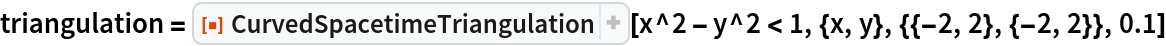Out=Show the causal graph:

 In:=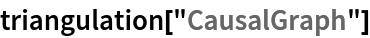Out=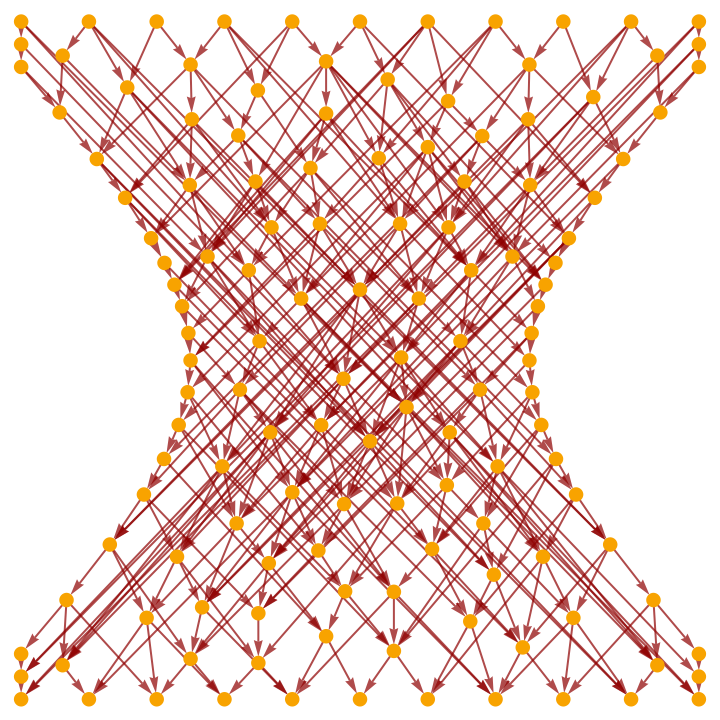Show the full causal graph (without transitive reduction):

 In:=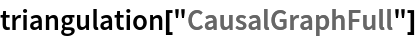Out=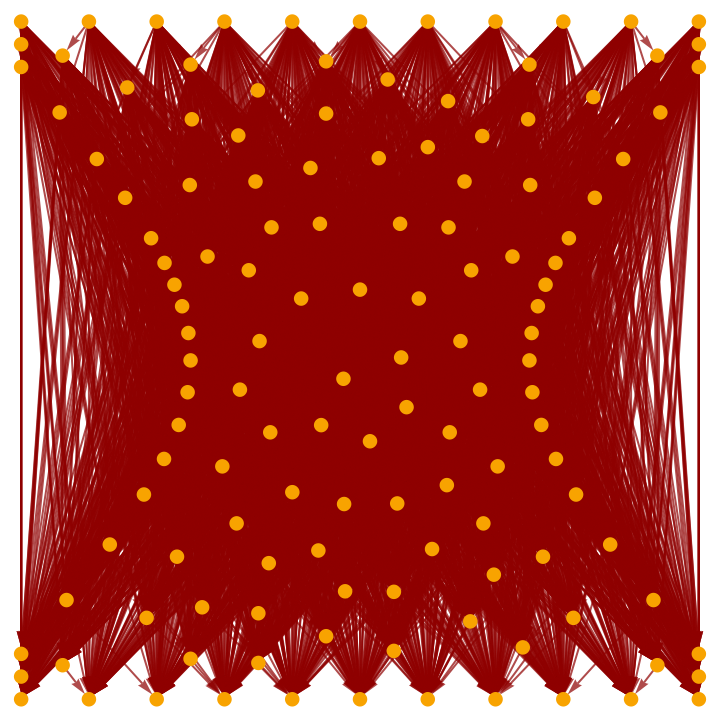Show the causal graph using only triangulation edges:

 In:=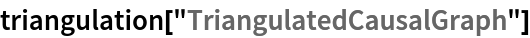Out=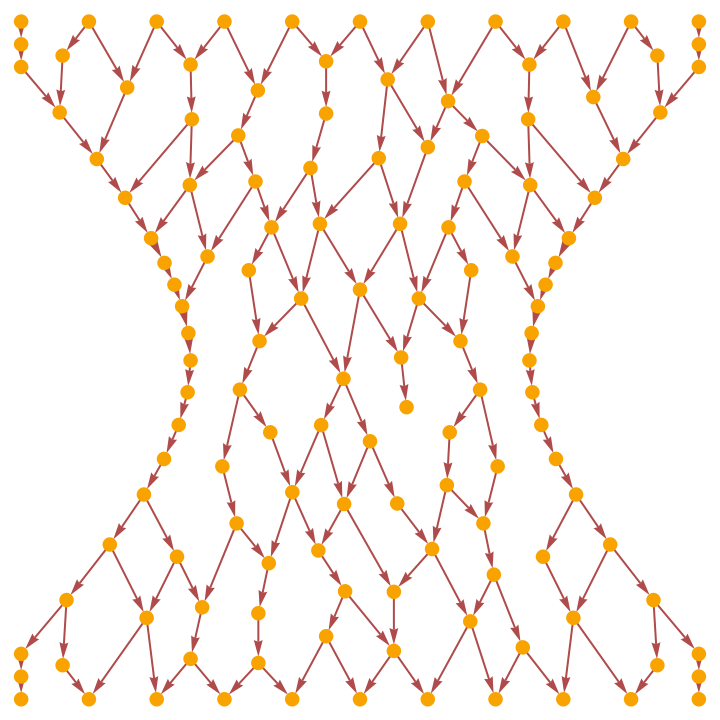Show the mesh region corresponding to the triangulated spacetime surface:

 In:=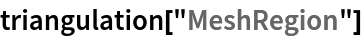Out=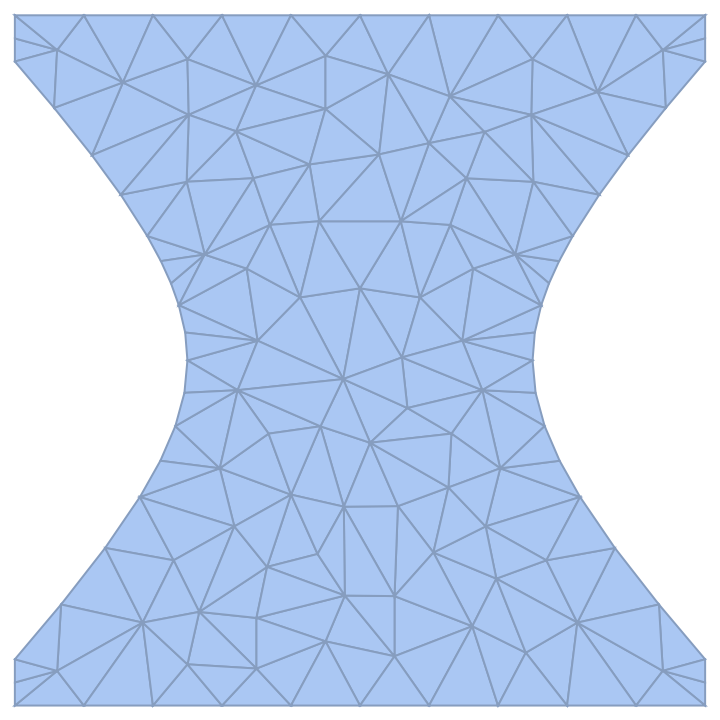Show the connectivity graph of this mesh region:

 In:=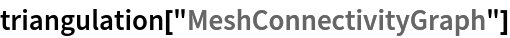Out=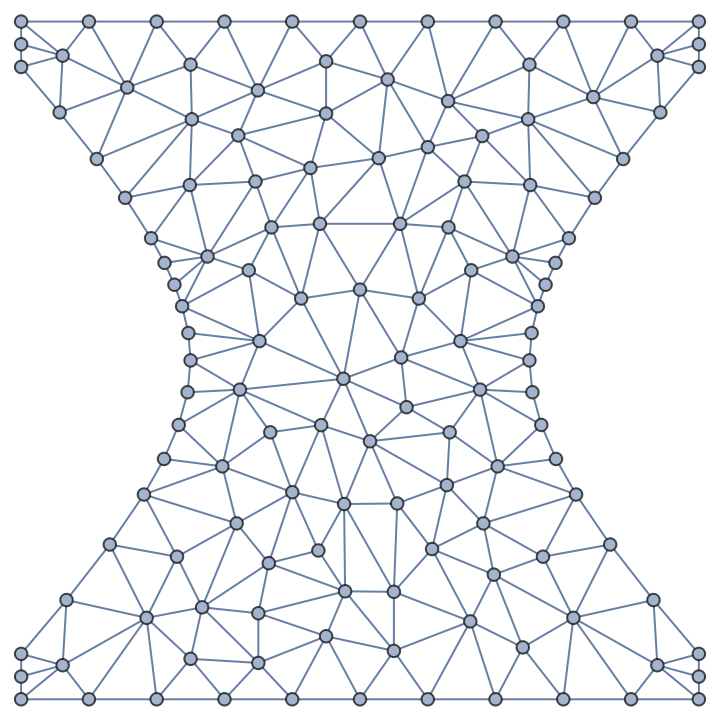Produce a triangulation of a 2+1-dimensional hyperboloidal region of spacetime (i.e. de Sitter space) into simplices of measure (volume) up to 1:

 In:=Out=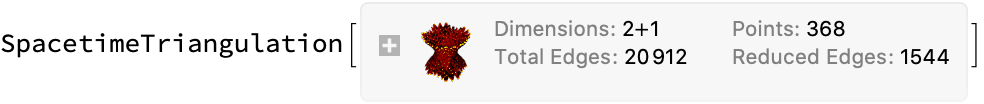Show the causal graph:

 In:=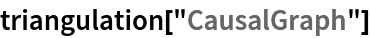Out=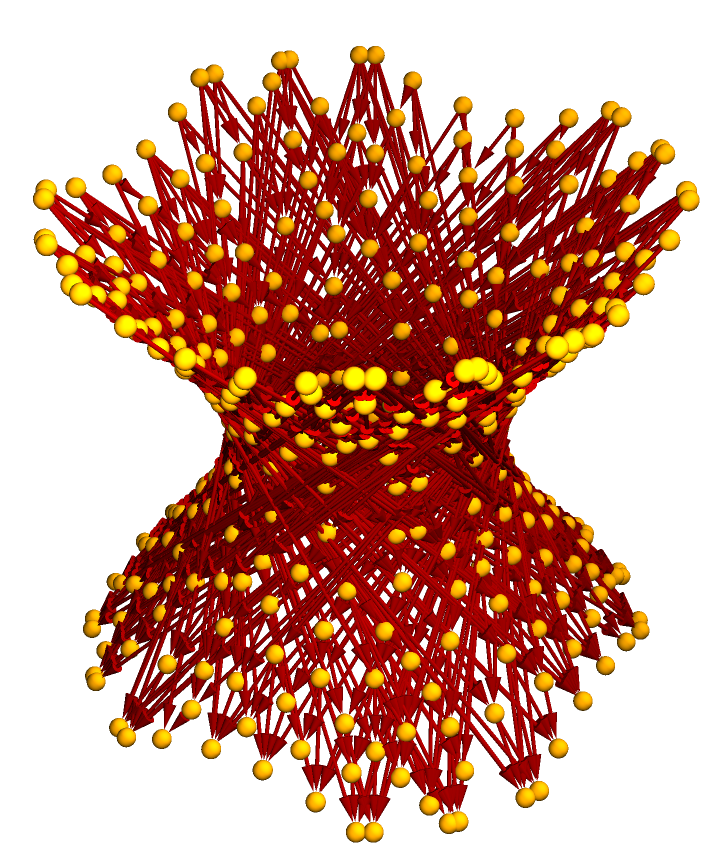Show the pure causal graph (with vertex coordinate information removed):

 In:=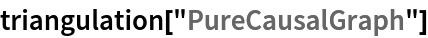Out=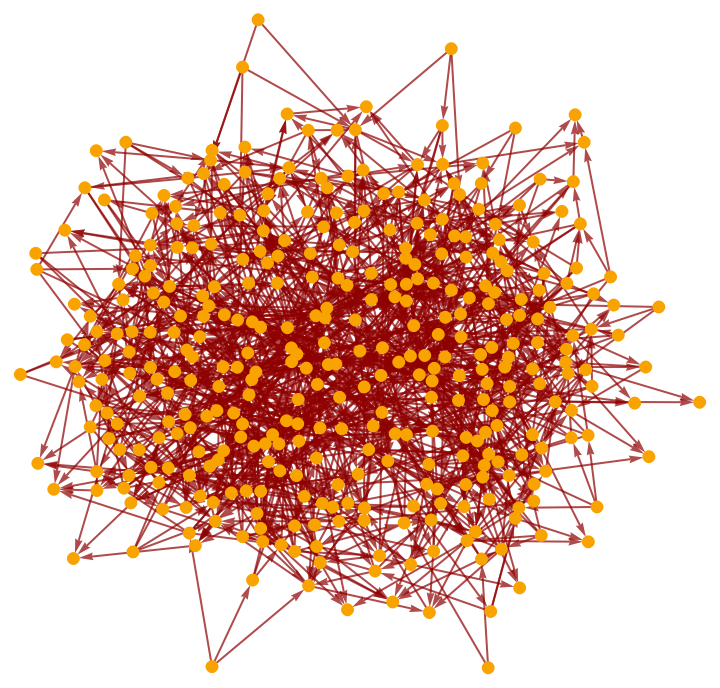Show the pure causal graph with a layered graph embedding:

 In:=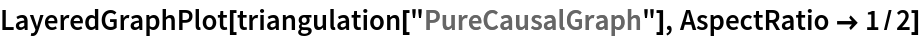Out=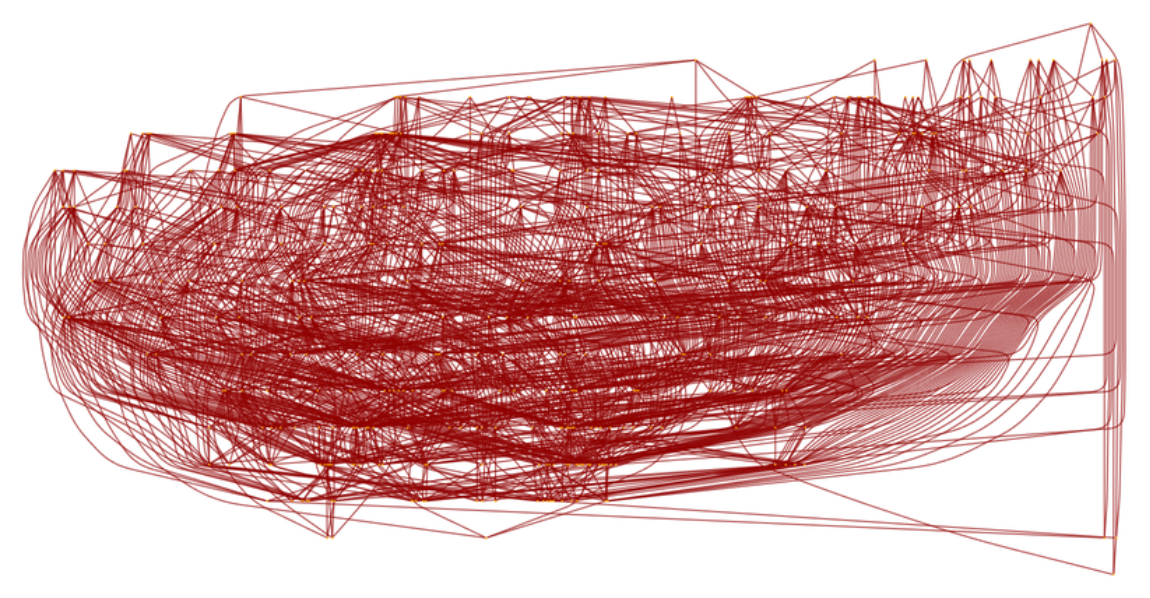Show only the pure triangulated causal graph (with vertex coordinate information removed):

 In:=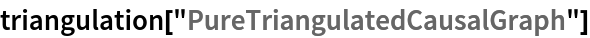Out=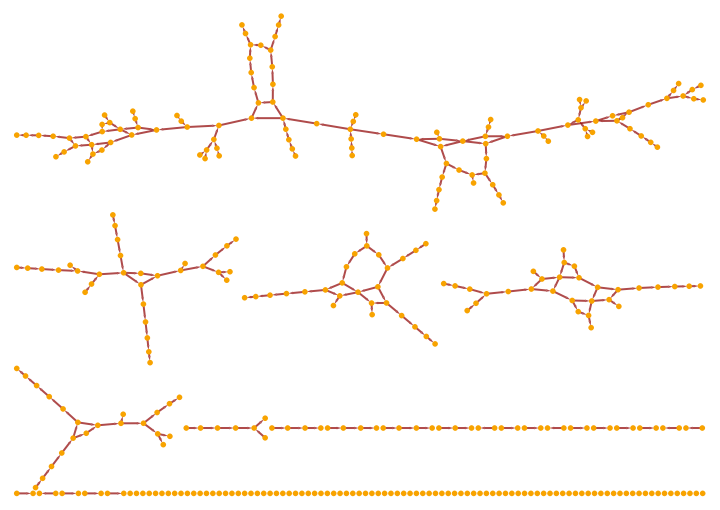Produce a triangulation of a 2+1-dimensional paraboloidal region of spacetime based on Flamm's paraboloid (related to the Schwarzschild geometry) into simplices of measure (volume) up to 0.1:

 In:=Out=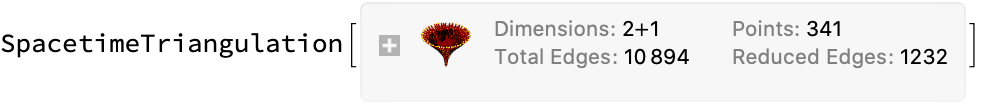Plot the positions of the points in the triangulation only (without causal edges):

 In:=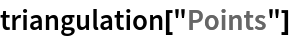Out=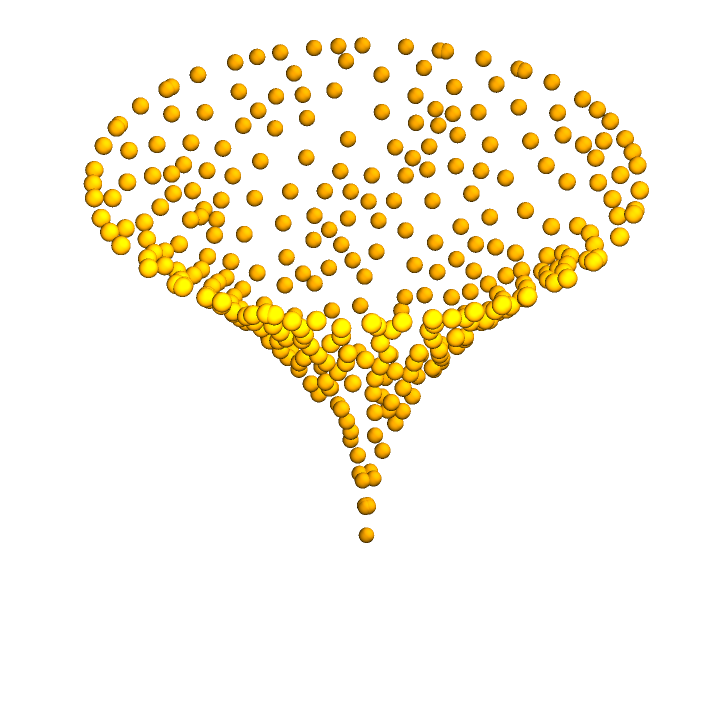Show the causal graph:

 In:=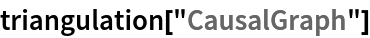Out=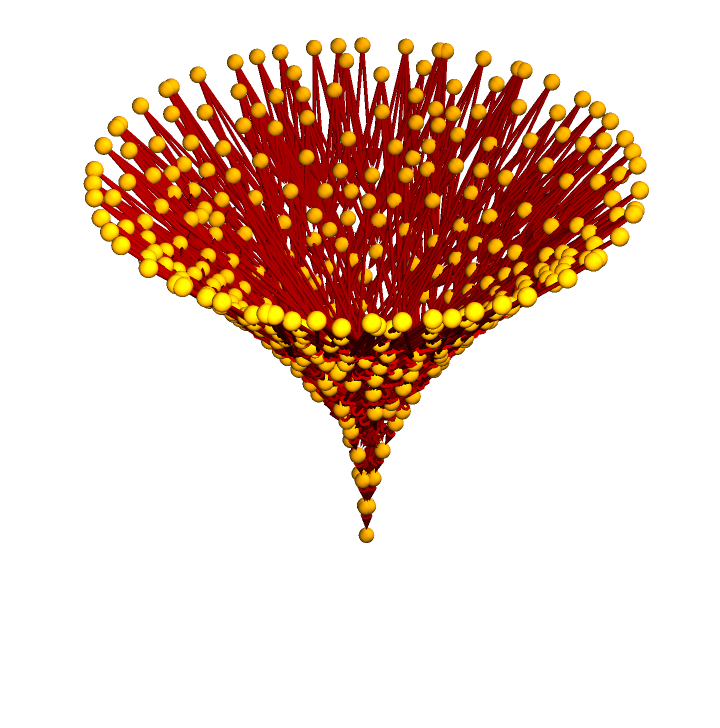By default, the region is restricted to the bounds {{-1,1},}:

 In:=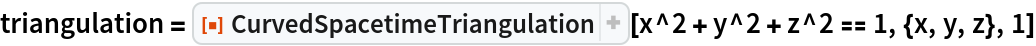Out=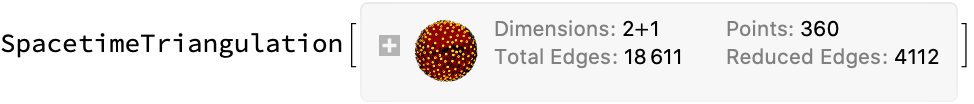Show the causal graph:

 In:=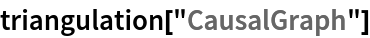Out=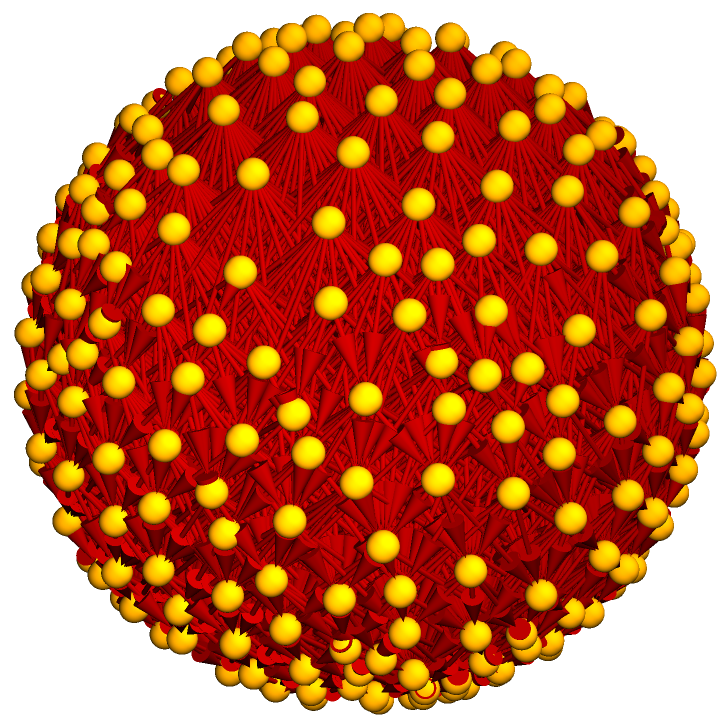### Scope (5)

Triangulations can be produced for 0+1-dimensional spacetimes:

 In:=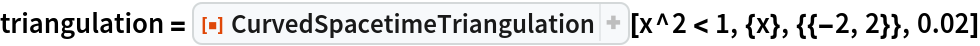Out=Show the pure causal graph:

 In:=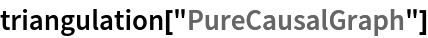Out=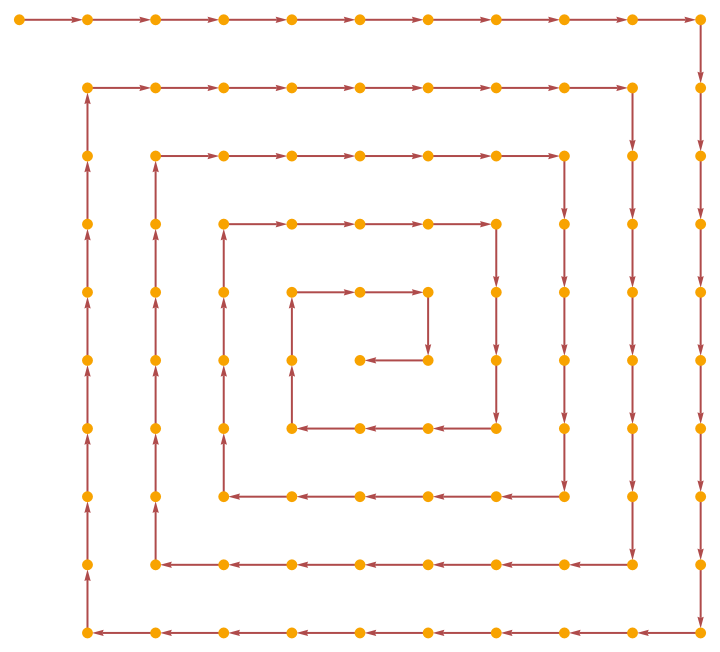Produce a triangulation of a 1+1-dimensional spacetime:

 In:=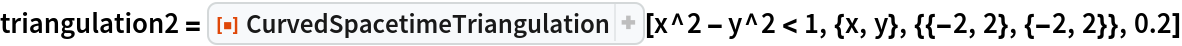Out=Show the causal graph:

 In:=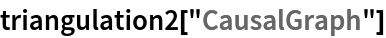Out=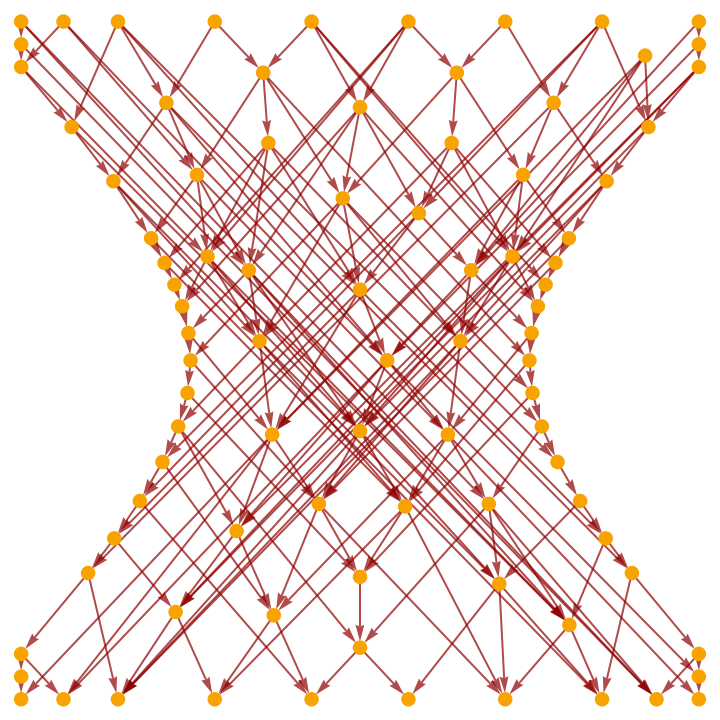Produce a triangulation of a 2+1-dimensional spacetime:

 In:=Out=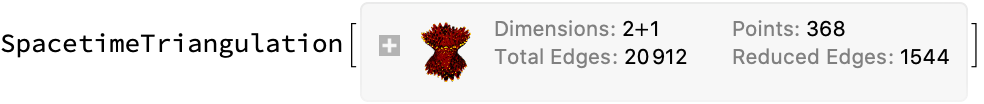Show the pure causal graph:

 In:=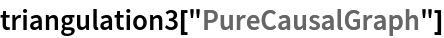Out=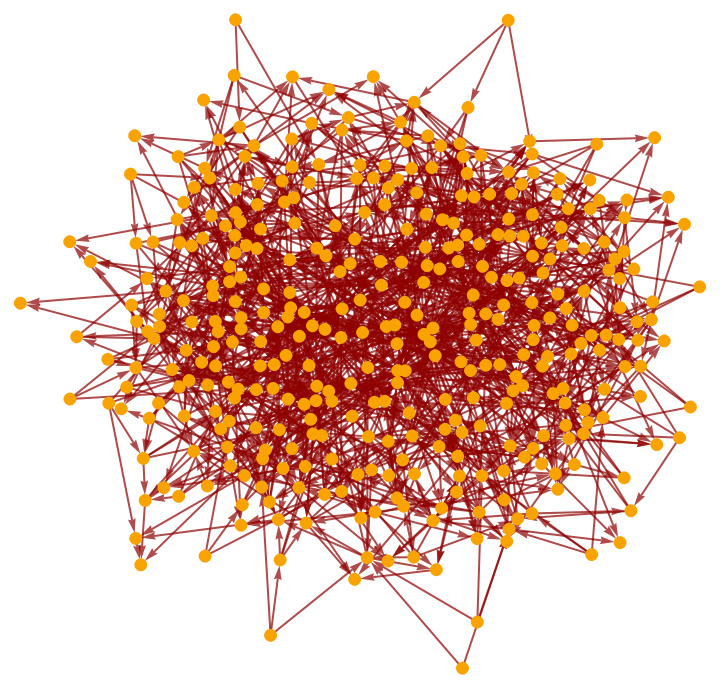Properties can be requested directly from CurvedSpacetimeTriangulation:

 In:=Out=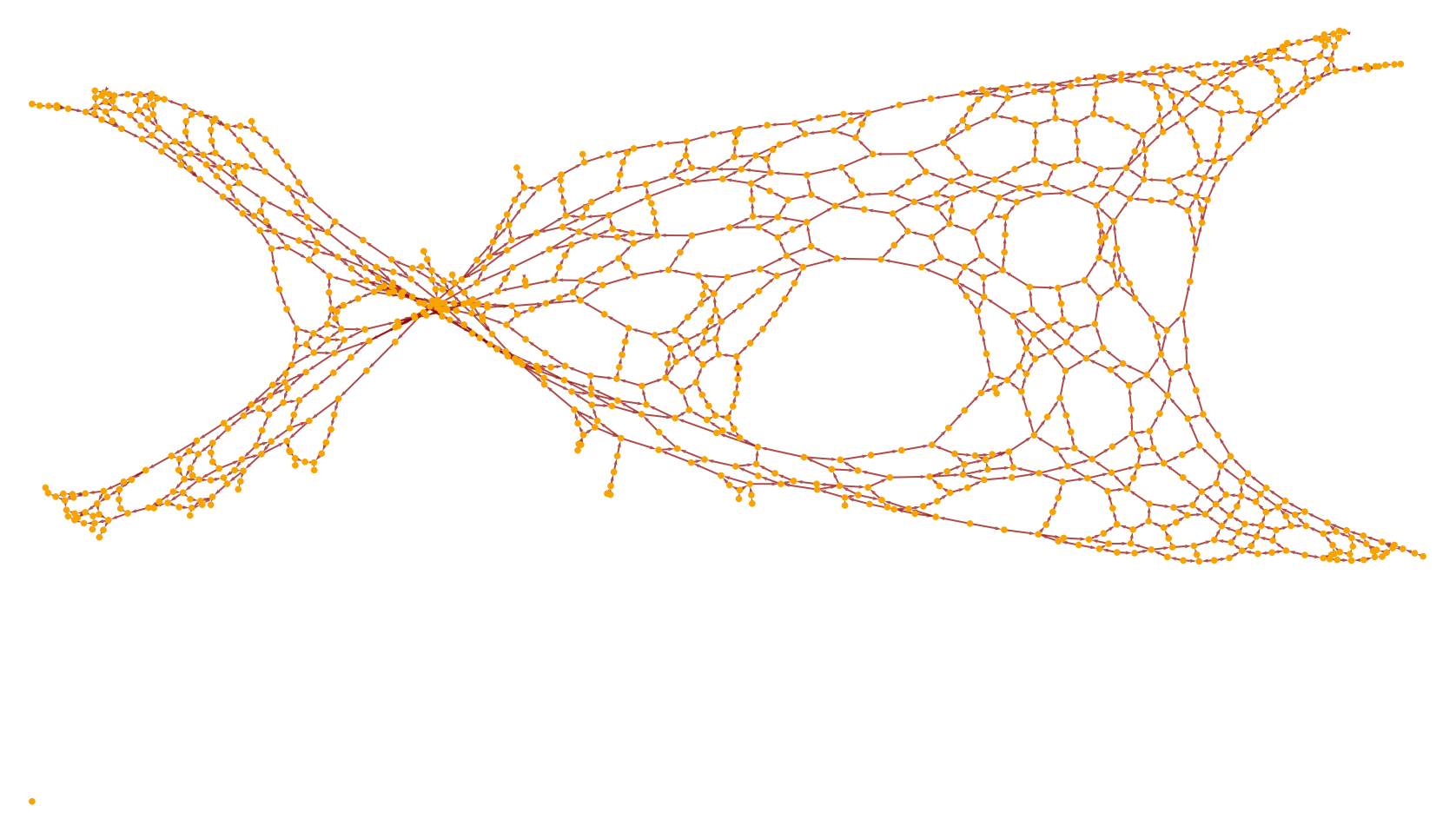Produce a triangulation of a 1+1-dimensional hyperbolic region of spacetime into simplices of measure (area) up to 0.1:

 In:=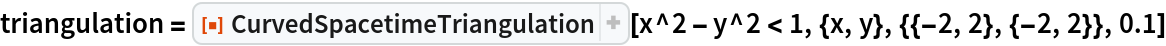Out=Show the complete list of properties:

 In:=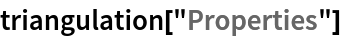Out=Show the full causal graph:

 In:=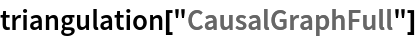Out=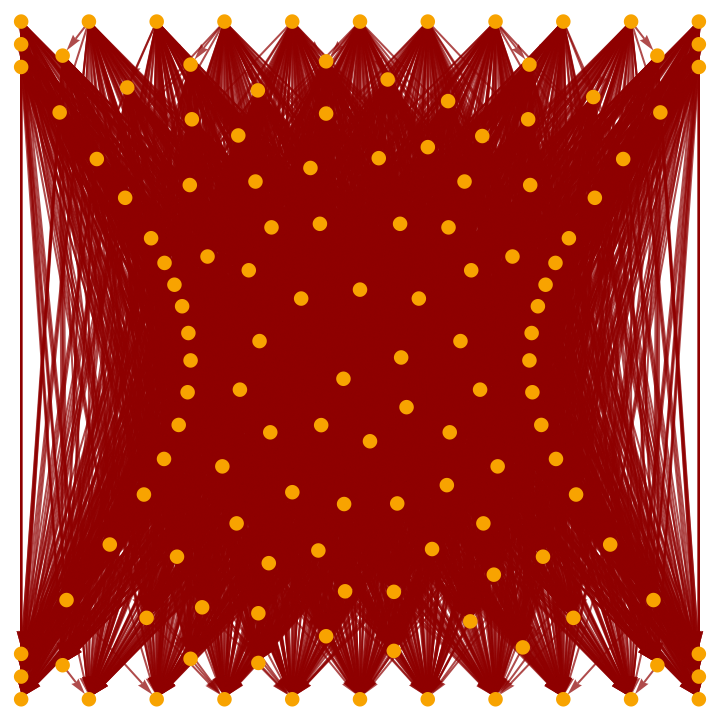Show the transitively-reduced causal graph:

 In:=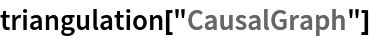Out=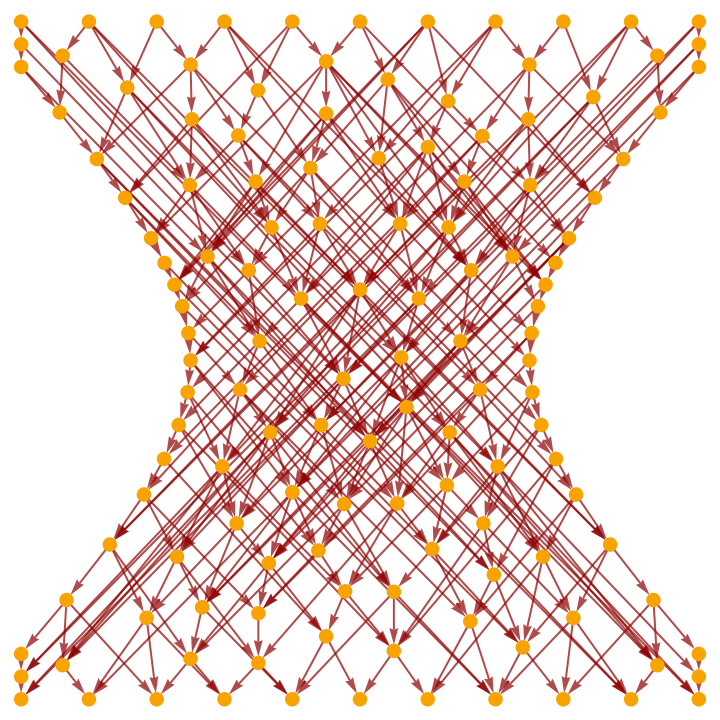Show the full causal graph using only triangulation edges:

 In:=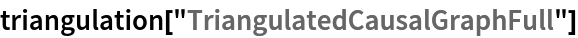Out=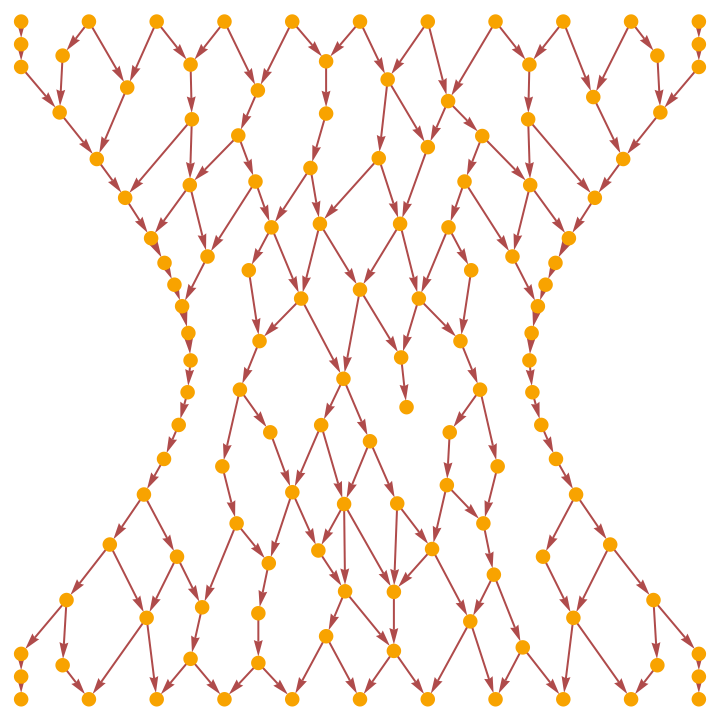Show the transitively-reduced causal graph using only triangulation edges:

 In:=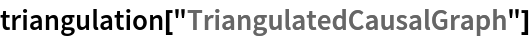Out=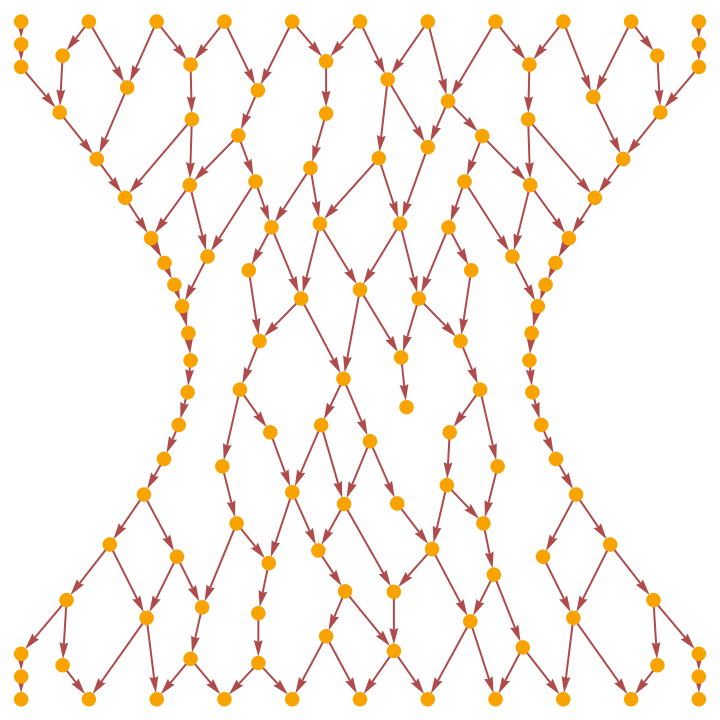Plot the positions of the points in the triangulation only (without causal edges):

 In:=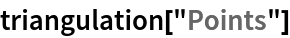Out=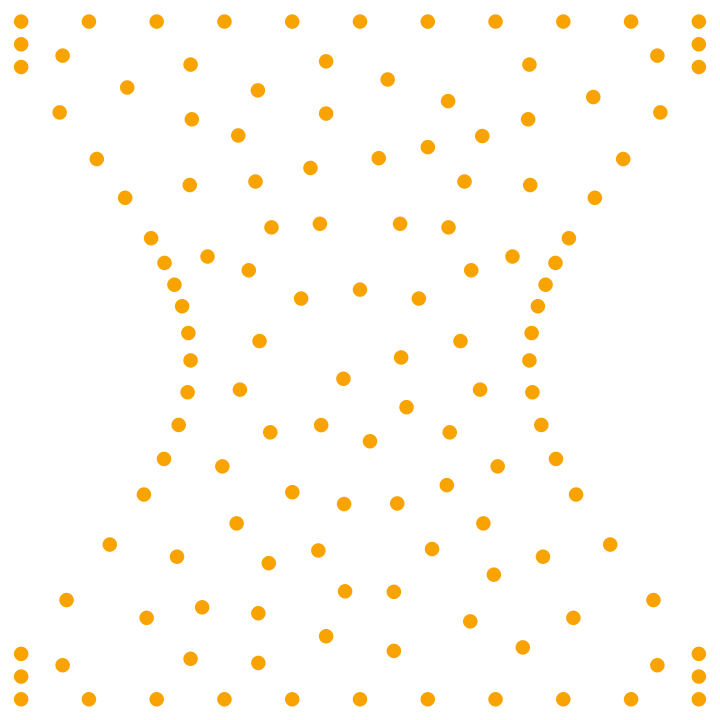Show a complete list of spacetime coordinates for the points in the triangulation (the last coordinate is always the time coordinate):

 In:=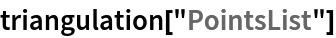Out=Show the number of points in the triangulation:

 In:=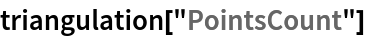Out=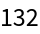Show the number of dimensions in the background spacetime:

 In:=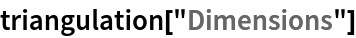Out=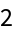Show the first 50 (undirected) edges in the triangulation of the spacetime surface:

 In:=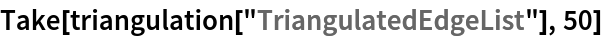Out=Show the total number of (undirected) edges in the triangulation of the spacetime surface:

 In:=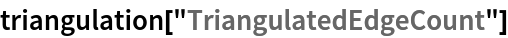Out=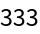Show the maximum measure (in this case, area) of the simplices in the triangulation:

 In:=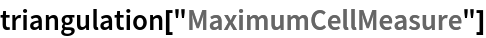Out=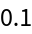Show the total number of causal edges:

 In:=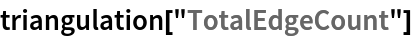Out=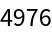Show the number of causal edges after transitive reduction:

 In:=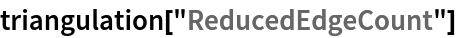Out=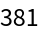Show the total number of causal edges appearing in the triangulation:

 In:=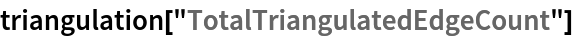Out=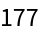Show the number of causal edges appearing in the triangulation after transitive reduction:

 In:=Out=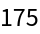Show the pure full causal graph (with vertex coordinate information removed):

 In:=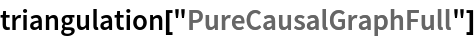Out=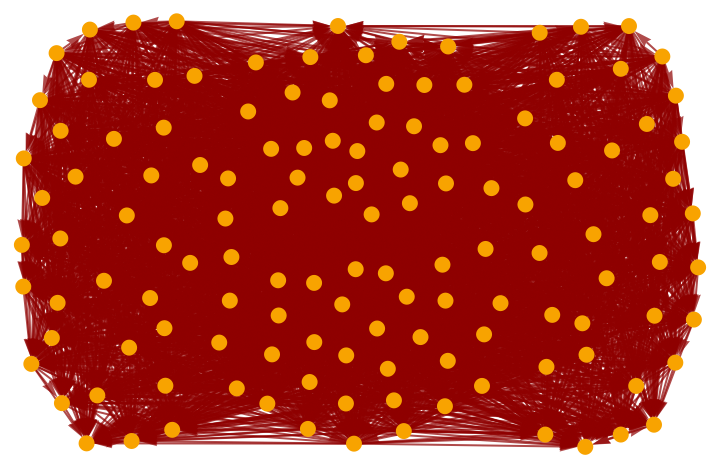Show the pure transitively-reduced causal graph (with vertex coordinate information removed):

 In:=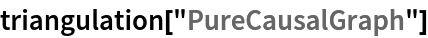Out=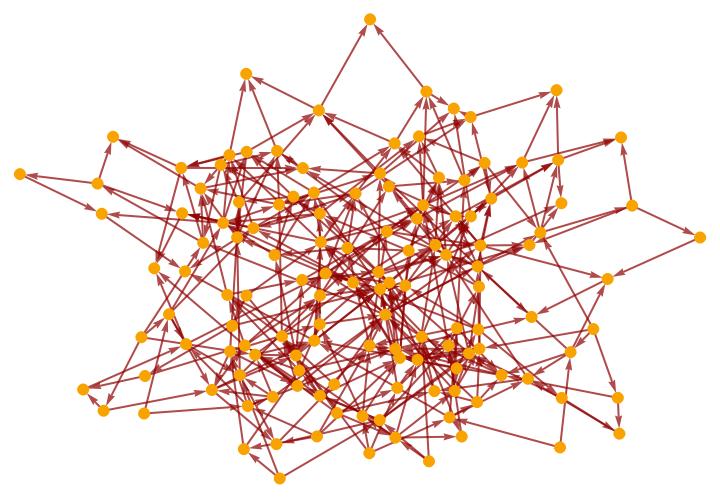Show the pure full causal graph using only triangulation edges (with vertex coordinate information removed):

 In:=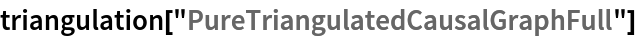Out=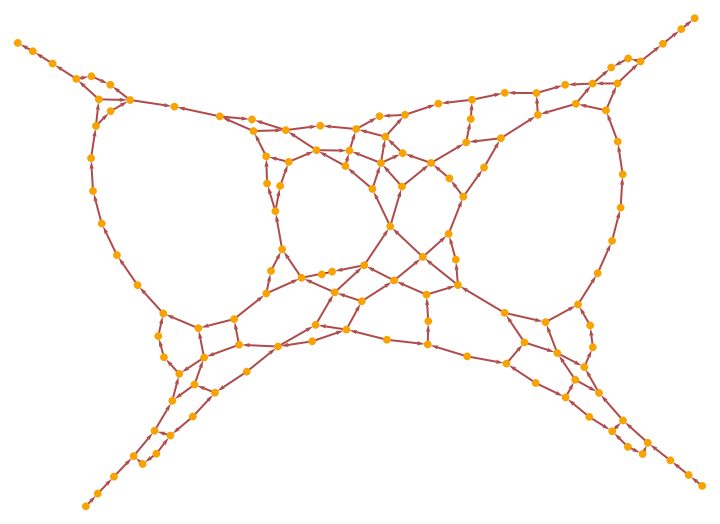Show the pure transitively-reduced causal graph using only triangulation edges (with vertex coordinate information removed):

 In:=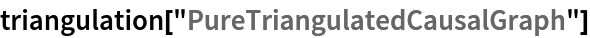Out=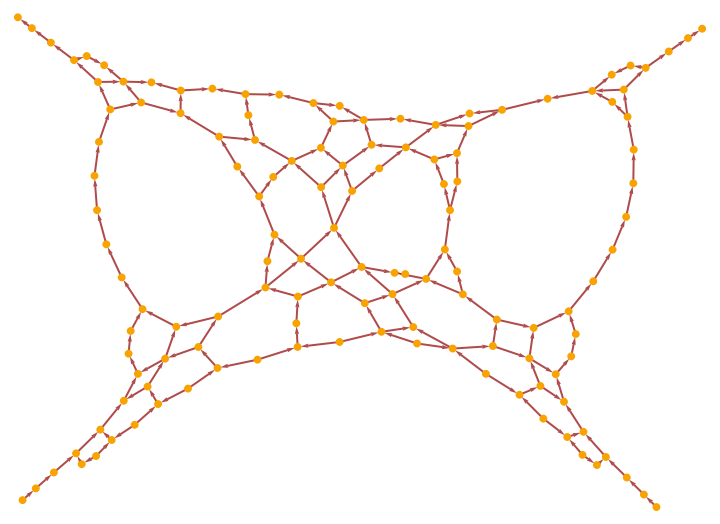Show the mesh region corresponding to the triangulated spacetime surface:

 In:=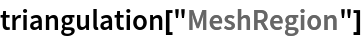Out=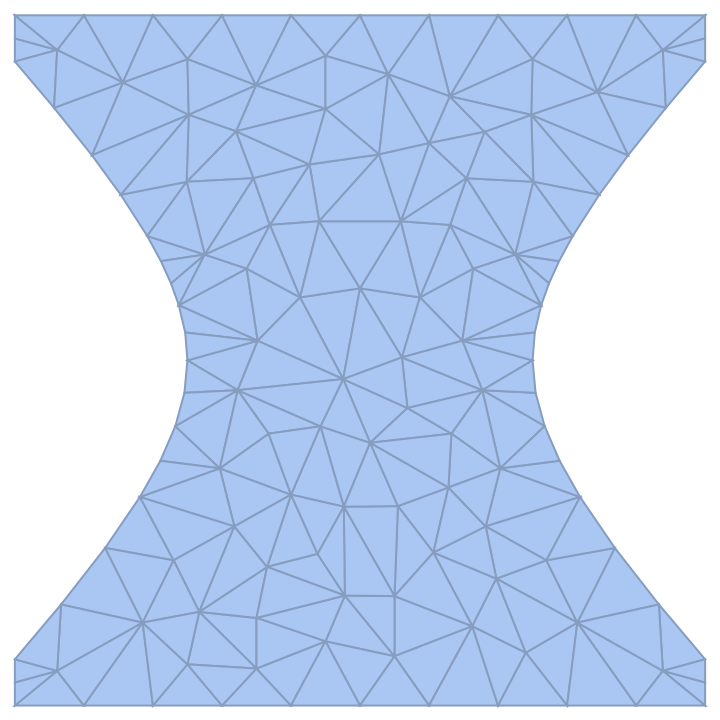Show the connectivity graph of this mesh region:

 In:=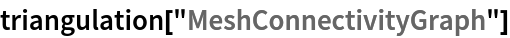Out=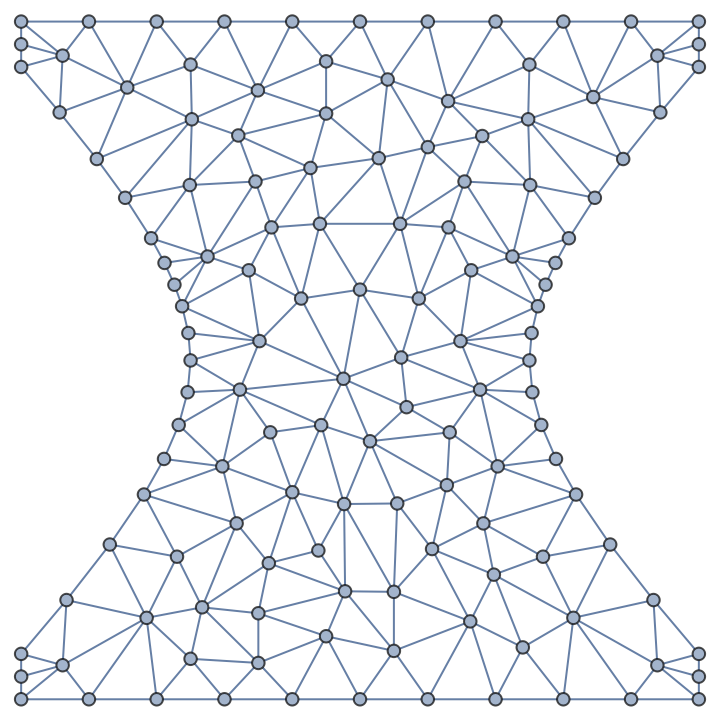Jonathan Gorard

## Version History

• 1.0.0 – 23 July 2021